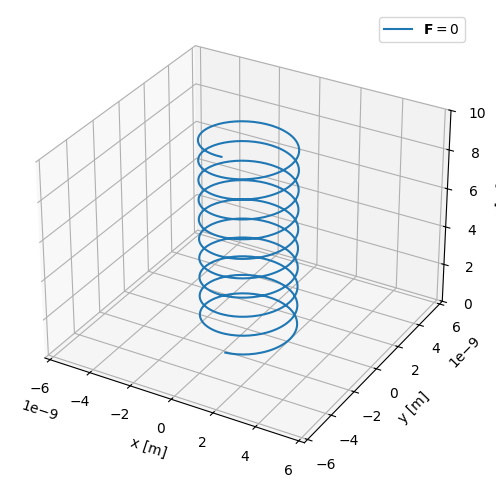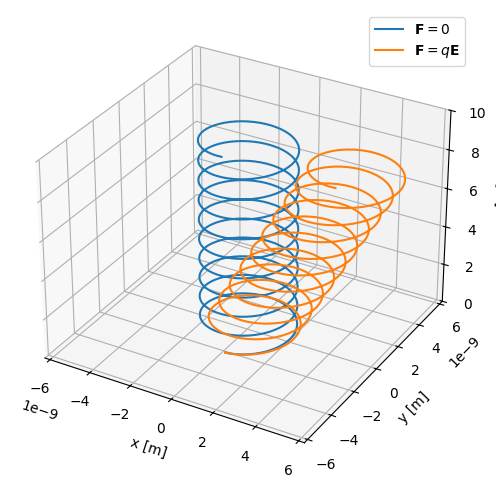:

%matplotlib inline

import astropy.units as u
import math
import matplotlib.pyplot as plt
import numpy as np

from plasmapy import formulary, particles


# Physics of the E×B drift

Consider a single particle of mass $$m$$ and charge $$q$$ in a constant, uniform magnetic field $$\mathbf{B}=B\ \hat{\mathbf{z}}$$. In the absence of external forces, it travels with velocity $$\mathbf{v}$$ governed by the equation of motion

$m\frac{d\mathbf{v}}{dt} = q\mathbf{v}\times\mathbf{B}$

which equates the net force on the particle to the corresponding Lorentz force. Assuming the particle initially (at time $$t=0$$) has $$\mathbf{v}$$ in the $$x,z$$ plane (with $$v_y=0$$), solving reveals

$v_x = v_\perp\cos\omega_c t \quad\mathrm{;}\quad v_y = -\frac{q}{\lvert q \rvert}v_\perp\sin \omega_c t$

while the parallel velocity $$v_z$$ is constant. This indicates that the particle gyrates in a circular orbit in the $$x,y$$ plane with constant speed $$v_\perp$$, angular frequency $$\omega_c = \frac{\lvert q\rvert B}{m}$$, and Larmor radius $$r_L=\frac{v_\perp}{\omega_c}$$.

As an example, take one proton p+ moving with velocity $$1\ m/s$$ in the $$x$$-direction at $$t=0$$:

:

# Initialize proton in uniform B field
B = 5 * u.T
proton = particles.Particle("p+")
omega_c = formulary.frequencies.gyrofrequency(B, proton)
v_perp = 1 * u.m / u.s


We can define a function that evolves the particle’s position according to the relations above describing $$v_x,v_y$$, and $$v_z$$. The option to add a constant drift velocity $$v_d$$ to the solution is included as an argument, though this drift velocity is zero by default:

:

def single_particle_trajectory(v_d=np.array([0, 0, 0])):
# Set time resolution & velocity such that proton goes 1 meter along B per rotation
T = 2 * math.pi / omega_c.value  # rotation period
v_parallel = 1 / T * u.m / u.s
dt = T / 1e2 * u.s

# Set initial particle position
x = []
y = []
xt = 0 * u.m
yt = -r_L

# Evolve motion
timesteps = np.arange(0, 10 * T, dt.value)
for t in list(timesteps):
v_x = v_perp * math.cos(omega_c.value * t) + v_d
v_y = v_perp * math.sin(omega_c.value * t) + v_d
xt += +v_x * dt
yt += +v_y * dt
x.append(xt.value)
y.append(yt.value)
x = np.array(x)
y = np.array(y)
z = v_parallel.value * timesteps

return x, y, z


Executing with the default argument and plotting the particle trajectory gives the expected helical motion, with a radius equal to the Larmor radius:

:

x, y, z = single_particle_trajectory()

:

fig = plt.figure(figsize=(6, 6))
ax.plot(x, y, z, label=r"$\mathbf{F}=0$")
ax.legend()
bound = 3 * r_L.value
ax.set_xlim([-bound, bound])
ax.set_ylim([-bound, bound])
ax.set_zlim([0, 10])
ax.set_xlabel("x [m]")
ax.set_ylabel("y [m]")
ax.set_zlabel("z [m]")
plt.show():

print(f"r_L = {r_L.value:.2e} m")

r_L = 2.09e-09 m


How does this motion change when a constant external force $$\mathbf{F}$$ is added? The new equation of motion is

$m\frac{d\mathbf{v}}{dt} = q\mathbf{v}\times\mathbf{B} + \mathbf{F}$

and we can find a solution by considering velocities of the form $$\mathbf{v}=\mathbf{v}_\parallel + \mathbf{v}_L + \mathbf{v}_d$$. Here, $$\mathbf{v}_\parallel$$ is the velocity parallel to the magnetic field, $$\mathbf{v}_L$$ is the Larmor gyration velocity in the absence of $$\mathbf{F}$$ found previously, and $$\mathbf{v}_d$$ is some constant drift velocity perpendicular to the magnetic field. Then, we find that

$F_\parallel = m\frac{dv_\parallel}{dt} \quad\mathrm{and}\quad \mathbf{F}_\perp = q\mathbf{B}\times \mathbf{v}_d$

and applying the vector triple product yields

$\mathbf{v}_d = \frac{1}{q}\frac{\mathbf{F}_\perp\times\mathbf{B}}{B^2}$

In the case where the external force $$\mathbf{F} = q\mathbf{E}$$ is due to a constant electric field, this is the constant $$\mathbf{E}\times\mathbf{B}$$ drift velocity:

$\boxed{ \mathbf{v}_d = \frac{\mathbf{E}\times\mathbf{B}}{B^2} }$

Built in drift functions allow you to account for the new force added to the system in two different ways:

:

E = 0.2 * u.V / u.m  # E-field magnitude
ey = np.array([0, 1, 0])
ez = np.array([0, 0, 1])
F = proton.charge * E  # force due to E-field

v_d = formulary.drifts.force_drift(F * ey, B * ez, proton.charge)
print("F drift velocity: ", v_d)
v_d = formulary.drifts.ExB_drift(E * ey, B * ez)
print("ExB drift velocity: ", v_d)

F drift velocity:  [0.04 0.   0.  ] m / s
ExB drift velocity:  [0.04 0.   0.  ] m / s


The resulting particle trajectory can be compared to the case without drifts by calling our previously defined function with the drift velocity now as an argument. As expected, there is a constant drift in the direction of $$\mathbf{E}\times\mathbf{B}$$:

:

x_d, y_d, z_d = single_particle_trajectory(v_d=v_d)

:

fig = plt.figure(figsize=(6, 6))
ax.plot(x, y, z, label=r"$\mathbf{F}=0$")
ax.plot(x_d, y_d, z_d, label=r"$\mathbf{F}=q\mathbf{E}$")

bound = 3 * r_L.value
ax.set_xlim([-bound, bound])
ax.set_ylim([-bound, bound])
ax.set_zlim([0, 10])
ax.set_xlabel("x [m]")
ax.set_ylabel("y [m]")
ax.set_zlabel("z [m]")
ax.legend()
plt.show():

print(f"r_L = {r_L.value:.2e} m")

r_L = 2.09e-09 m

Of course, the implementation in our single_particle_trajectory() function requires the analytical solution for the velocity $$\mathbf{v}_d$$. This solution can be compared with that implemented in the particle stepper notebook. It uses the Boris algorithm to evolve the particle along its trajectory in prescribed $$\mathbf{E}$$ and $$\mathbf{B}$$ fields, and thus does not require the analytical solution.